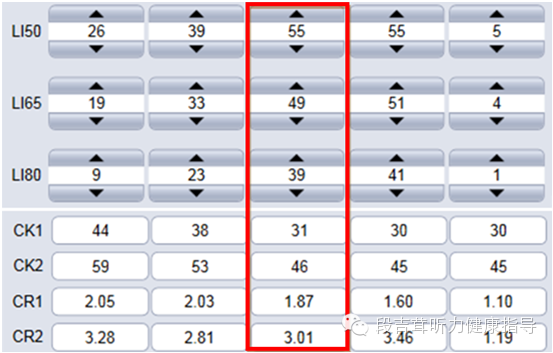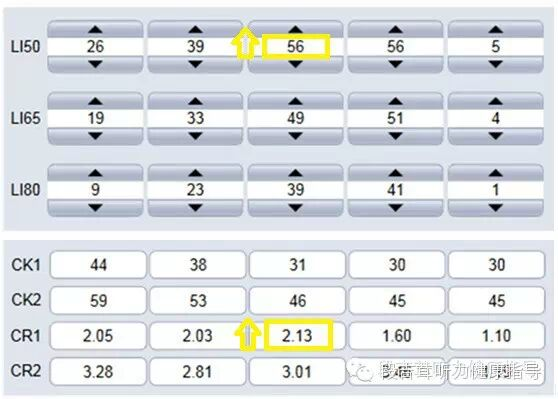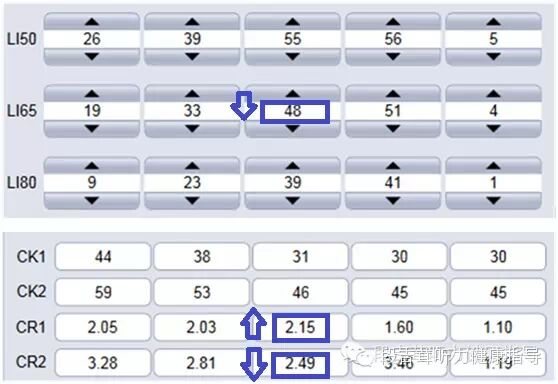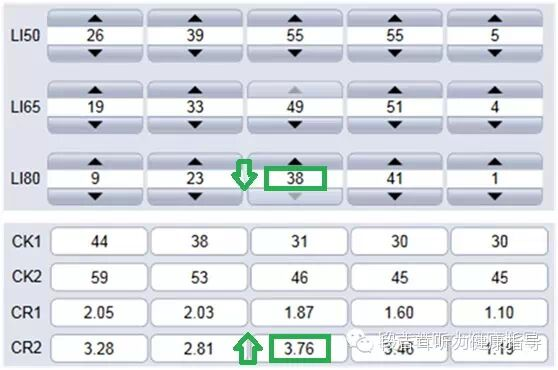# 如何通过改变助听器的声音大小来调节压缩参数？

2015-02-02 11:17来源: 互联网 编辑:济南助听器  点击:CK1表示第一个压缩拐点，CK2表示第二个压缩拐点；CR1表示第一个压缩比，CR2表示第二个压缩比。

CK1=31，CK2=46；

CR1=1.87，CR2=3.01CK1=31CK2=46；

CR1=2.13CR2=3.01CK1=31CK2=46；

CR1=2.15CR2=2.49CK1=31CK2=46；

CR1=1.87CR2=3.76

• 轻声、中等声和大声并不专指50dB65dB80dB的声音，还可以使用其他相应的声压级表示。

• 不同验配软件的调节界面不同，调节选项也不同，尽管如此，上述增益与压缩比的关系是保持一致的。

• 虽然单拐点压缩系统和双拐点压缩系统有所不同，但调节增益依然可以影响压缩参数。